International Journal of Fluid Mechanics & Thermal Sciences
Volume 2, Issue 2, August 2016, Pages: 10-15

Meshing Effect on the Hydrodynamic Structure Around Water Darrieus Rotor

Ibrahim Mabrouki, Zied Driss*, Ahmed Samet, Mohamed Salah Abid

Laboratory of Electro-Mechanic Systems (LASEM), National School of Engineers of Sfax (ENIS), University of Sfax, Sfax, Tunisia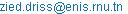(Z. Driss)

*Corresponding author

Ibrahim Mabrouki, Zied Driss, Ahmed Samet, Mohamed Salah Abid. Meshing Effect on the Hydrodynamic Structure Around Water Darrieus Rotor. Science Journal of Energy Engineering. Vol. 2, No. 2, 2016, pp. 10-15. doi: 10.11648/j.ijfmts.20160202.11

Received: April 12, 2016; Accepted: April 18, 2016; Published: July 5, 2016

Abstract: Turbulent free surface flows are encountered in many hydraulic and water resources engineering problems. In this work, the CFD code "Fluent" is used to study the meshing effect on the hydrodynamic structure around water Darrieus rotor. This code is based on solving steady Navier-Stokes equations by a finite volume discretization model. The numerical approach used is the multi reference frame (MRF) model. The validation of our computer model is done by the comparison with experimental anterior results.

Keywords: Darrieus Rotor, Meshing Effect, Hydrodynamic Structure, CFD, RANS

Contents

1. Introduction

The computer results consist on presentation of the velocity field, the velocity magnitude, the static pressure, the dynamic pressure, the turbulent kinetic energy, the dissipation rate of turbulent kinetic energy, the turbulent viscosity and the magnitude vorticity. These results offer local information about the flow around the turbine inside the duct and improve that the axial velocity value is more effective with refinement of the mesh. To verify our computer simulations, the axial velocity was compared with the experimental results. The good comparison between these results indicates the validity of our computer method.

2. Numerical Approach

In this application, we have conducted a numerical investigation of Darrieus rotor for low-head hydropower generation. The three-dimensional fluid flow is analyzed using the Navier-Stokes equations. The computational method used is based on a finite volume discretization. The computer results consist on presentation of the velocity field, the velocity magnitude, the static pressure, the dynamic pressure, the turbulent kinetic energy, dissipation rate of turbulent kinetic energy, the turbulent viscosity and the magnitude vorticity. These results offer local information about the flow around the turbine inside the duct and prove that the axial velocity value is more effective with refinement of the mesh.

2.1. Geometry System and Boundary Conditions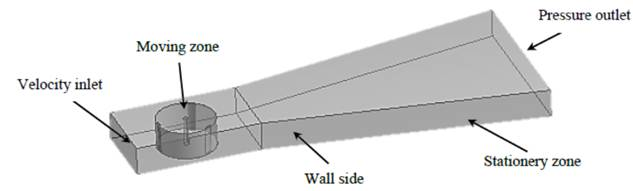Figure 1. Boundary Conditions.

The boundary conditions are presented in figure 1 using "GAMBIT" software. In the present study, the velocity inlet is equal to Vi = 1 m.s-1 and the pressure outlet is equal to p=101325 Pa. The compartment near the turbine presents a rotating moving zone area defined by ω = 25.61 rpm.

2.2. Meshing

To study the meshing effect, we have created four mesh sizes. The total number of cells are equal to 13821 in the first case (Figure 2.a), 330 309 in the second case (Figure 2.b), 956 054 in the third case (Figure 2.c), and 1 700 000 in the fourth case (Figure 2.d).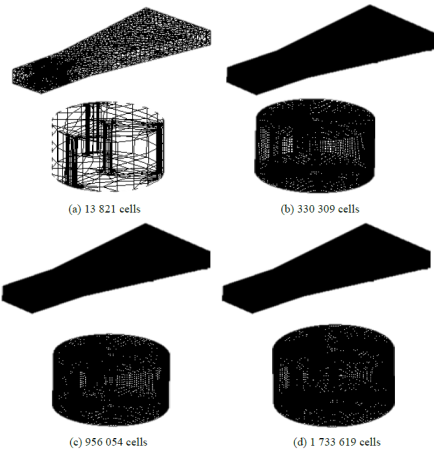Figure 2. 3-D views of the meshing.

3. Numerical Results

The numerical results are obtained by the application of multi-reference frame model. The test section equipped with water Darrieus rotor in which we have imposed a constant rotational speed ω = 25.61 rad.s-1. The turbulent ﬂow is deﬁned by the Reynolds number Re = 3.8 106. Particularly, we are interested to plot the results for tow mesh resolutions, the first with total of cells equal 13 821 and the second with 1 733 619.

3.1. Velocity Fields

Figures 3 and 4 show the distribution of the velocity fields in the test section. The considered planes are defined by z=0 m and y=0 m. According to these results, it is clear that the velocity vectors reach its maximum values in the small gaps between the rotor and the duct walls. Around the water Darrieus rotor, a rotating movement of the fluid has been observed. The recirculation zone of the fluid remains away from the rotor. Therefore, energy is transmitted to the rotor movement. Around the blades, the maximum value of velocity fields is obtained. It is about 3.5 m.s-1with 1 733 619 cells, and 3.7 m.s-1 with 13 821 cells. This fact is due to the special shapes of the blades. In the downstream of the rotor, the velocity value keeps decreasing until the out of the test section. Then, a sharp increase has been noted through the divergent angle where the velocity values are recorded in the lateral walls of the duct. Globally, similar results are obtained with the different meshing sizes. However, the difference is localized in the duct extremity and around the rotor. This difference is due to the difference between the sizes of grid.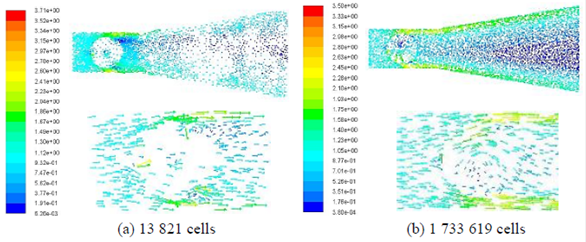Figure 3. Velocity fields distribution.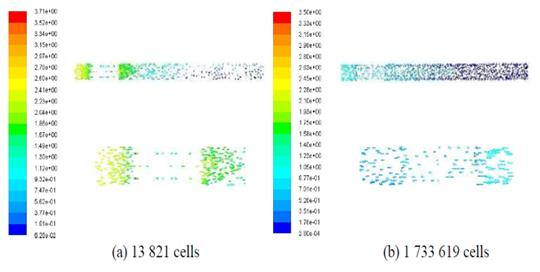Figure 4. Distribution of the velocity field in the longitudinal plane defined by y=0 m.

3.2. Magnitude Velocity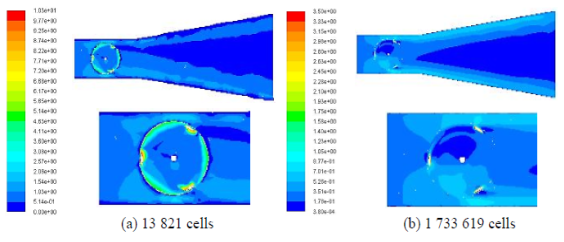Figure 5. Distribution of the magnitude velocity in the longitudinal plane defined by z=0 m.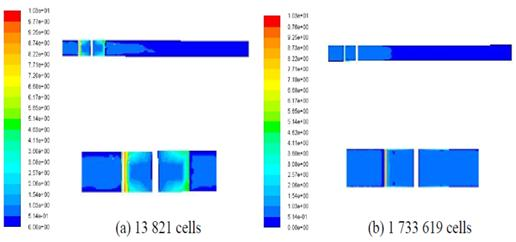Figure 6. Distribution of the magnitude velocity in the longitudinal plane defined by y=0 m.

Figures 5 and 6 present receptively the distribution of the magnitude velocity in the longitudinal and longitudinal planes defined by z=0 m and y=0 m. From these results, a first view shows that the magnitude velocity is found weak in the inlet of duct. It is indeed governed by the boundary condition value of the inlet velocity witch is equal to 1 m.s-1.While approaching to the water Darrieus rotor, the velocity field is affected by the geometric configuration. In fact, a flow acceleration appears in the gap between the rotor and the blades walls. This fact is confirmed in the longitudinal plane defined by z=0 m. In the downstream of the rotor, the magnitude velocity value keeps decreasing until the out of the test section. Then, a sharp increase has been noted through the duct where the velocity values are recorded in the lateral walls of the duct. This fact is confirmed in the longitudinal section plane defined by y=0 m. Globally, different results are obtained with the different meshing sizes. However, the difference is localized in the duct extremity and in the rotating area around the blades. This fact is due to the mesh sizes.

3.3. Static Pressure

Figures 7 and 8 show the distribution of the static pressure in the test section equipped by the water Darrieus rotor. According to these results, a considerable increase of static pressure has been observed in the upstream of the blades. However, a depression zones appear in the downstream. In the longitudinal section plane defined by z=0 m, it has been noted that the pressure increases slightly in the first part of the test section and it decreases rapidly in the rotor downstream. Near the blades a compression and depression zones appeared in the intrados and extrados respectively.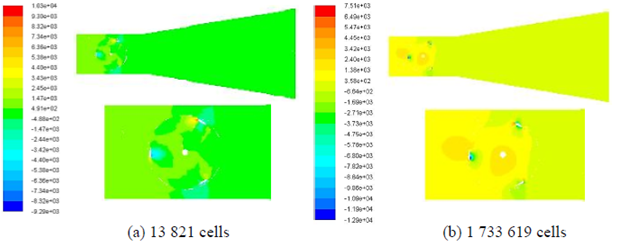Figure 7. Distribution of the static pressure in the longitudinal plane defined by z=0 m.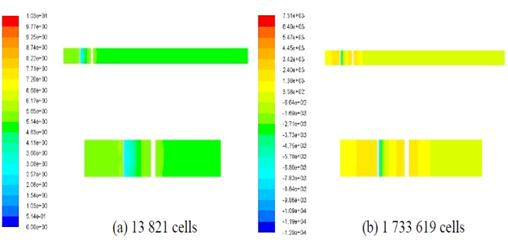Figure 8. Distribution of the static pressure in the longitudinal plane defined by y=0 m.

3.4. Dynamic Pressure

Figures 9 and 10 illustrate the distribution of the dynamic pressure in the test section equipped by the water Darrieus rotor. In the longitudinal planes defined respectively by z=0 m and y=0 m, the dynamic pressure is found to be weak in the inlet of the test section. It increases gradually through the gaps between the walls duct and the rotor. A depression zone is recorded in the rotating area around the axis and in the downstream of the rotor. The maximum value is obtained in the gap between the rotor and the duct walls. Under these conditions, the dynamic pressure is equal to 2.58103 Pa with a 13821 cells, and to 2.4103 Pa with a 1 733 619 cells. This difference is due to the grid sizes modification.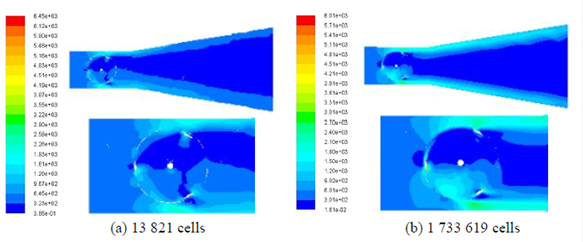Figure 9. Distribution of the dynamic pressure in the longitudinal plane defined by z=0 m.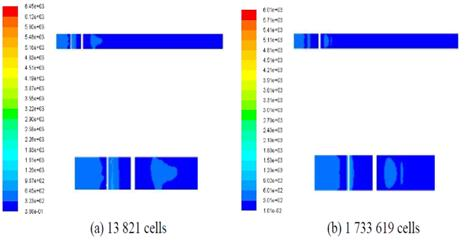Figure 10. Distribution of the dynamic pressure in the longitudinal plane defined by y=0 m.

3.5. Turbulent Kinetic Energy

Figures 11 and 12 show the distribution of the turbulent kinetic energy over the entire test section equipped by the water Darrieus rotor. From these results, it is clear that the turbulent kinetic energy is very weak in the test section except in the area surrounding the rotor. The distribution of the turbulent kinetic energy shows the increase of the energy in the interior zone of the rotor. From a first glance, it has been observed a difference between distributions of the turbulent kinetic energy for each meshing sizes. This difference is located in the rotating area, exactly around the blades. The maximum value of the turbulent kinetic energy is recorded around the blades. Under these conditions, the turbulent kinetic energy is equal to 0.479 m2.s-2with a 13821 cells and to 0.495 m2.s-2 with a 1 733619 cells.

Globally, similar results are obtained with the different mesh. However, the difference is localized in the rotating area around the blades walls.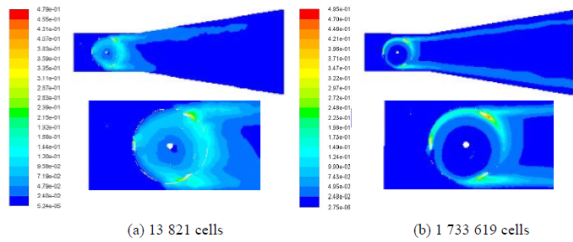Figure 11. Distribution of the turbulent kinetic energy in the longitudinal plane z=0 m.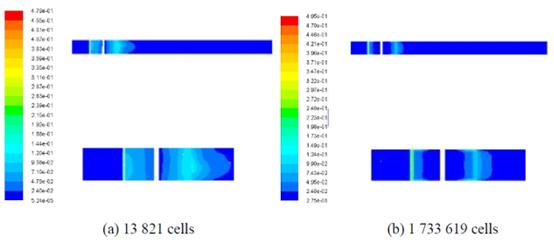Figure 12. Distribution of the turbulent kinetic energy in the longitudinal plane y=0 m.

3.6. Dissipation Rate of the Turbulent Kinetic Energy

Figures 13 and 14 show the distribution of the dissipation rate of the turbulent kinetic energy in the test section equipped by the water Darrieus rotor in the longitudinal and longitudinal planes defined respectively by z=0 m and y=0 m. In the case of 13 821 cells, the extension of the wake zone characteristic of the maximal values of dissipation rate of the turbulent kinetic energy is very limited, but in the case of 1 733 619 cells, it has been noted that the dissipation rate is located near the blades walls in the rotating zone. This fact is due to the transformation of the turbulent kinetic energy into heat. The distribution of the dissipation rate of the turbulent kinetic energy in the longitudinal plane shows that a brutal increase of the dissipation rate is more important in the superior and inferior parts of the blades with a 1 733 619 cells.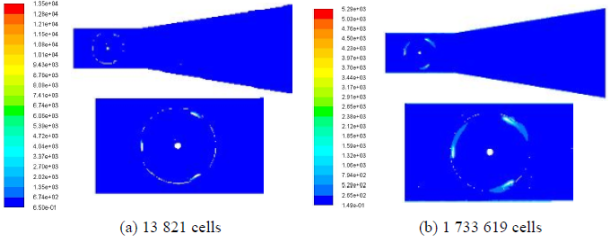Figure 13. Distribution of the dissipation rate of the turbulent kinetic energy in the longitudinal plane defined by z=0 m.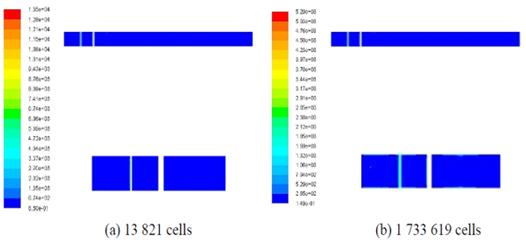Figure 14. Distribution of the dissipation rate of the turbulent kinetic energy in the longitudinal plane defined by y=0 m.

3.7. Turbulent Viscosity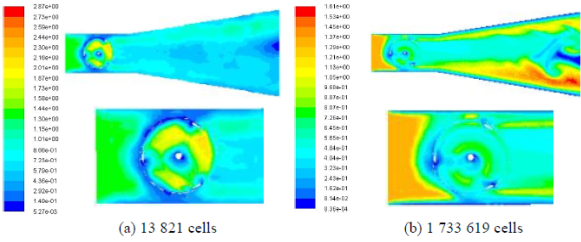Figure 15. Distribution of the turbulent viscosity in the longitudinal plane defined by z=0 m.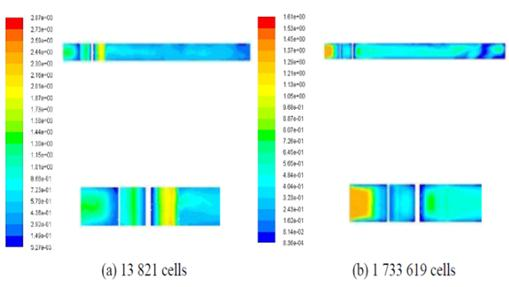Figure 16. Distribution of the turbulent viscosity in the longitudinal plane defined by y=0 m.

Figures 15 and 16 present the distribution of the turbulent viscosity throughout the test section equipped by the water Darrieus rotor. According to these results, it has been noted that the turbulent viscosity is very important in the upstream of the rotating area. A rapid decrease has been observed around and downstream of the rotor. Indeed, the turbulent viscosity is found to be weak in the duct interior; but it increases considerably in the duct’s walls. The maximum viscosity values are situated in the duct’s outlet. This fact is confirmed in the longitudinal and longitudinal planes defined by z=0 m and y=0 m respectively. Under these conditions, the maximum value of turbulent viscosity lacalizated in the downstream of the duct is equal to 1.61 kg/m.s with a 1 733619 cells and to 2.01 kg/m.s with a 13821 cells. Globally, similar results are obtained with the different meshing sizes. However, the difference is localized in the upstream of the rotating area and near the wall duct in the downstream.

3.8. Magnitude Vorticity

Figures 17 and 18 show the distribution of the magnitude vorticity in the test section equipped by the water Darrieus rotor. According to these results, the vorticity is found to be near to zero almost everywhere except in the region around the rotor. The greatest vorticity values are reached in the internal and external sides of the blades. The distribution of the vorticity in the longitudinal plane defined by y=0 m shows that the maximum vorticity values are recorded in the superior and inferior parts of the blades. The distribution in the longitudinal plane defined by z=0 m shows another maximum value zone in the convex surface of the blades. Globally, similar results are obtained with the different meshing sizes. However, the difference is localized in the extremity of the blades walls.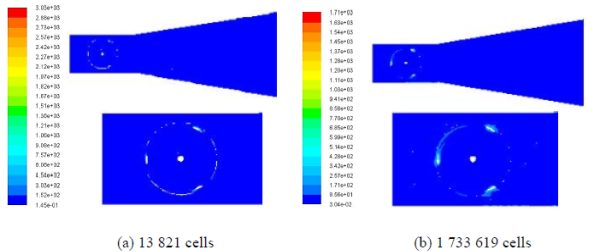Figure 17. Distribution of the magnitude vorticity in the longitudinal plane defined by z=0 m.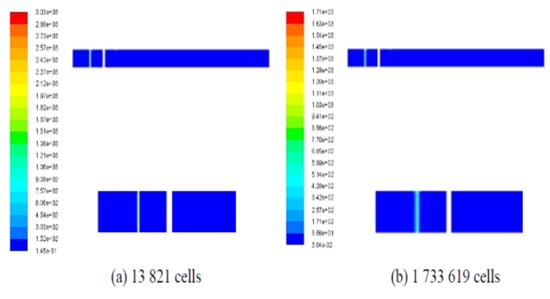Figure 18. Distribution of the magnitude vorticity in the longitudinal plane defined by y=0 m.

4. Comparison with Experimental Results

Figure 19 shows the different profiles of the dimensional axial velocity as function of 2y/D position for different cells sizes. These results present the superposition of the numerical results gathered from the CFD code "FLUENT" and the experimental results of Matsushita et al. . The different velocity profiles seem to have the same appearance but the velocity values depends on the cell size. Indeed, the greater the cell size gets the more, the gap between numerical and experimental results is large. The best results regarding precision are found for 956 054 and 1733619 cells. Also, it has been noted that the resolution time increases with the decrease of the size of mesh cells. The best result regarding precision and time is found for 956 054 cells. This choice leads to a better result with regards to the precision and the resolution time.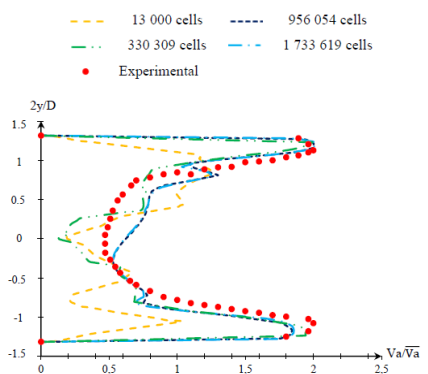Figure 19. Velocity profiles.

5. Conclusions

In this paper, we have studied the meshing effect on the hydrodynamic structure around water Darrieus rotor. The computer results consist on presentation of the velocity field, the magnitude velocity, the static pressure, the dynamic pressure and the turbulence characteristics. For the local results, it has been observed that the difference is localized in the duct extremity and in the rotating area around the blades. For the velocity profiles, the same appearance has been obtained but the velocity values depend on the cell size. In fact, the greater the cell size gets the more the gap between numerical and experimental results is large. Indeed, it has been noted that the resolution time increases with the decrease of the size of mesh cells.

In the future, we propose to study others numerical parameters to improve the numerical simulations.

References

1. Brochier, G., Fraunié, P., Béguier, C., Paraschivoiu, I., 1986, Water channel experiments of dynamic stall on Darrieus wind turbine blades, Journal of Propulsion and Power, 2(5), 445-449.
2. Templin, R.J., 1974, Aerodynamic performance theory for the NRC vertical axis wind turbine, National Research Council of Canada Report, LTR-LA-160.
3. Strickland, J.H., 1975, The Darrieus turbine: a performance prediction model using multiple stream tubes. Sandia Laboratories Report. SAND75-0431.
4. Loth, J.L., McCoy, H., Optimization of Darrieus turbine with an upwind and downwind momentum model, Journal of Energy 1983, 7(4), 313-318.
5. Paraschivoiu, I., Delclaux, F., 1983, Double multiple streamtubes. Model with recent improvement. Journal of Energy, 7(3), 250-255.
6. Glauert, H., 1976, Airplane propellers. In: Durand WF, editor. Div. L, Aerodynamic theory. Berlin: Springer, 169-360.
7. Masson, C., Leclerc, C., Paraschivoiu, I., 1998, Appropriate dynamic-stall models for performance predictions of VAWTs with NLF blades, International Journal of Rotating Machinery, 4(2), 129-139.
8. Mabrouki, I., Driss, Z., and Abid, M.S., 2014, Experimental investigation of the height effect of water Savonius rotors, International journal of mechanics and application, 4(1), 8-12.
9. Mabrouki, I., Driss, Z., and Abid, M.S, "Influence of the hight on Characteristics of Savonius Hydraulic Turbine," International Conference on Mechanics and Energy ICME’2014, March 18-20, 2014, Monastir, TUNISIA.
10. Mabrouki, I., Driss, Z., and Abid, M.S., "Computer modeling of 3D turbulent free surface flow in a water channel with and without obstacle," International Conference on Mechanics and Energy ICME’2014, March 18-20, 2014, Monastir, TUNISIA.
11. Driss, Z., Mlayeh, O., Driss, D., Maaloul, M, Abid, M.S., 2014, Numerical simulation and experimental validation of the turbulent flow around a small incurved Savonius wind rotor, Energy, 74, 506-517.
12. Daisuke, M., Kusuo, O., Satoshi, W., Akinori, F., 2008, Simplified structure of ducted Darrieus-type hydro turbine with narrow intake for extra-low head hydropower utilization, Journal of fluid science and technology, 3, 387-397.

 Contents 1. 2. 2.1. 2.2. 3. 3.1. 3.2. 3.3. 3.4. 3.5. 3.6. 3.7. 3.8. 4. 5.
Article ToolsAbstractPDF(1836K)